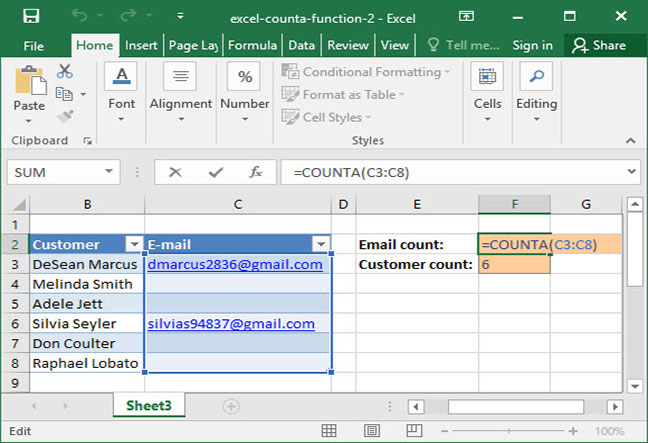# Using Excel's COUNTA Function

If you're familiar with Excel's COUNT function, you know how to count the number of cells in a range that contain numerical values. But what if you want to count the number of cells in a range that contain any value — including strings of text? For this task, unfortunately, COUNT falls short.

To solve the problem, Excel has a similar, equally-useful function: COUNTA. COUNTA works exactly like COUNT, except that it includes all cells, not just those with numerical values. COUNTA includes cells with text, errors, and numbers — anything that isn't blank.

If you aren't yet familiar with the COUNT function, learning about it can be helpful in advance of this tutorial. Take a look at our overview of the COUNT function for more information.

## The basic COUNTA function

Like COUNT, COUNTA is very easy to learn:

`=COUNTA(value_or_range, value_or_range_2 (optional)...)`

When fed one or more ranges of cells or values, COUNTA will output the number of cells or values that are non-blank — meaning that they include either numerical values, strings of text, or errors.

Here's an example of COUNTA used with static inputs:

`=COUNTA(5, "Boston")Output: 2`

The above formula outputs the number 2, because there are 2 arguments provided, both of which are singular inputs (a number and a string of text).

Note that COUNTA also works with errors and empty text strings:

`=COUNTA(5, "Seven", 10, #N/A, "")Output: 5`

This formula spits out the number 5, because there are 5 static inputs provided. Note that even the empty text string ("") is counted as non-blank.

## COUNTA with a range

Of course, counting the number of static inputs to a COUNTA formula isn't particularly useful. The function becomes much more versatile when we use it to count the number of non-blank cells in a range.

In the following example, a SnackWorld analyst has a list of customer names paired with e-mail addresses. Some of the customers have left their e-mail addresses, but some haven't — so not every name is matched to an address. The analyst wants to know how many e-mail addresses and customers are on the list, respectively. So, she writes the following formula:`=COUNTA(C3:C8)Output: 2`

This formula evaluates to 2, because there are 2 e-mail addresses present on the list (and thus 2 non-blank cells in the range C3:C8.

The same formula works to count the number of customers on the range B3:B8:

`=COUNTA(B3:B8)Output: 6`

## When to use Excel's COUNTA function

Now that you know how to use COUNTA, you can probably think of many potential applications. Here are a few ideas to get you started:

• Count the number of customers on a customer list;
• Count the number of transactions made in a given time period;
• Find how many tests have been submitted and scored by students; or
• Calculate the number of days of data available in a data set.

Questions or comments? Let us know below!

## Save an hour of work a day with these 5 advanced Excel tricks

Work smarter, not harder. Sign up for our 5-day mini-course to receive must-learn lessons on getting Excel to do your work for you.

• How to create beautiful table formatting instantly...
• Why to rethink the way you do VLOOKUPs...
• Plus, we'll reveal why you shouldn't use PivotTables and what to use instead...